﻿ 一种改进的基于UKF的单站无源定位方法

# 一种改进的基于UKF的单站无源定位方法An Improved Algorithm Based on UKF for Single Station Passive Location

Abstract: Single station passive location is an important function of electronic reconnaissance device. However, the existing methods either refer to complicated nonlinear equations, or need the station to move long time. For the device which is restricted to real time response, low power dissipation, small volume, these methods are not suitable. Aim at this problem, an improved algorithm for single station passive location is proposed. First, triangulation is executed to acquire rough estimation, then optimizing the estimation based on UKF. Simulation results demonstrate that the proposed algorithm can get the location in time, and converge to real position.

1. 引言

2. 单站无源定位方法

2.1. 定位模型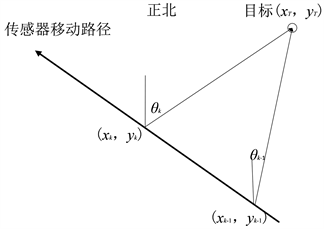Figure 1. Geometric diagram of passive positioning of single station

2.2. 三角交叉定位

$\left\{\begin{array}{l}\left({y}_{T}-{y}_{k-1}\right)tg{\theta }_{k-1}={x}_{T}-{x}_{k-1}\\ \left({y}_{T}-{y}_{k}\right)tg{\theta }_{k}={x}_{T}-{x}_{k}\end{array}$ (1)

$AX=Z$ (2)

$\begin{array}{l}A=\left[\begin{array}{cc}1& -tg{\theta }_{k-1}\\ 1& -tg{\theta }_{k}\end{array}\right],\\ X=\left[\begin{array}{c}{x}_{T}\\ {y}_{T}\end{array}\right],\\ Z=\left[\begin{array}{c}-{x}_{k-1}+{y}_{k-1}tg{\theta }_{k-1}\\ -{x}_{k}+{y}_{k}tg{\theta }_{k}\end{array}\right]\end{array}$ (3)

$X={\left({A}^{T}A\right)}^{-1}{A}^{T}Z$ (4)

2.3. UKF滤波

$\left[\begin{array}{c}{x}_{T,k}\\ {y}_{T,k}\end{array}\right]=\left[\begin{array}{cc}1& 0\\ 0& 1\end{array}\right]\left[\begin{array}{c}{x}_{T,k-1}\\ {y}_{T,k-1}\end{array}\right]$ (5)

${\theta }_{k}=\mathrm{arctan}\left(\frac{{y}_{T}-{y}_{k}}{{x}_{T}-{x}_{k}}\right)+{\eta }_{k}$ (6)

UT的具体过程如下：

1) 计算2n + 1个Sigma点及其权值：

$\begin{array}{l}{X}_{i}=\left\{\begin{array}{ll}\stackrel{¯}{X},\hfill & i=0\hfill \\ \stackrel{¯}{X}+\sqrt{\left(n+1\right){P}_{X}},\hfill & i=1,2,\cdots ,n\hfill \\ \stackrel{¯}{X}-\sqrt{\left(n+1\right){P}_{X}},\hfill & i=n+1,n+2,\cdots ,2n\hfill \end{array}\\ {w}_{i}^{m}=\left\{\begin{array}{ll}\frac{\lambda }{n+\lambda },\hfill & i=0\hfill \\ \frac{1}{2\left(n+\lambda \right)},\hfill & i=1,2,\cdots ,2n\hfill \end{array}\\ {w}_{i}^{c}=\left\{\begin{array}{ll}\frac{\lambda }{n+\lambda }+\left(1-{\alpha }^{2}+\beta \right),\hfill & i=0\hfill \\ \frac{1}{2\left(n+\lambda \right)},\hfill & i=1,2,\cdots ,2n\hfill \end{array}\end{array}$ (7)

2) 计算Sigma点通过非线性函数f()的结果：

${Y}_{i}=f\left({X}_{i}\right),\text{\hspace{0.17em}}\text{\hspace{0.17em}}i=0,1,\cdots ,2n$ (8)

$\begin{array}{l}\stackrel{¯}{Y}=\underset{i=0}{\overset{2n}{\sum }}{w}_{i}^{m}{Y}_{i}\\ {P}_{Y}=\underset{i=0}{\overset{2n}{\sum }}{w}_{i}^{c}\left({Y}_{i}-\stackrel{¯}{Y}\right){\left({Y}_{i}-\stackrel{¯}{Y}\right)}^{T}\end{array}$ (9)

2.4. 算法流程

1) 启动定位功能；

2) 平台移动一定距离后，进行三角交叉定位，获取初始定位点；

3) 以初始定位点作为UKF滤波起始估计值；

4) 由状态滤波估计值产生Sigma点；

5) 根据滤波方程，迭代更新目标状态估计，即目标位置估计值。

3. 仿真分析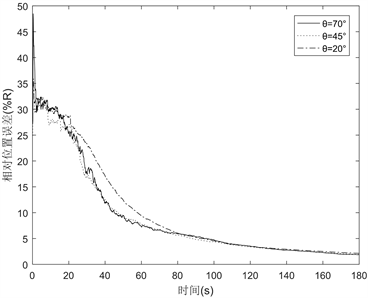(a) R = 30 km, σ = 1˚, T = 0.1 s, v = 50 m/s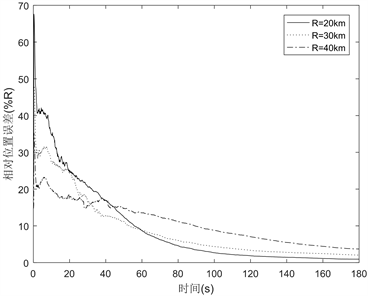(b) θ = 60˚, σ = 1˚, T = 0.1 s, v = 50 m/s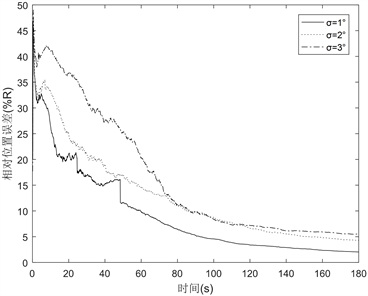(c) R = 30 km, θ = 60˚, T = 0.1 s, v = 50 m/s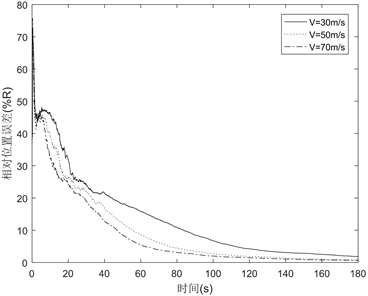(d) R = 20 km, θ = 60˚, σ = 1˚, T = 0.1 s

Figure 2. Analysis diagram of simulation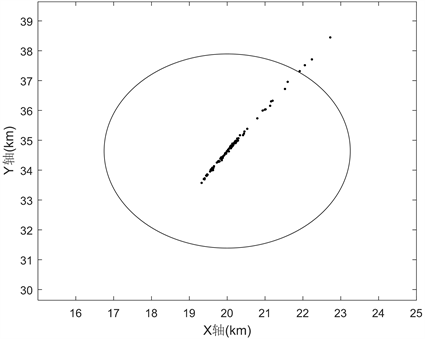Figure 3. Estimated location of single-station target (1)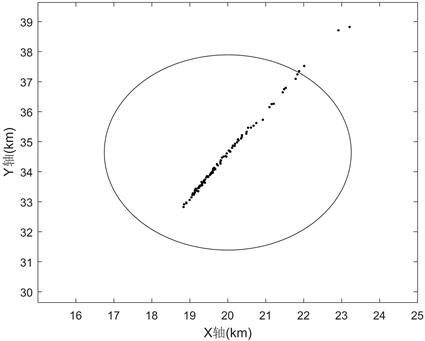Figure 4. Estimated location of single-station target (2)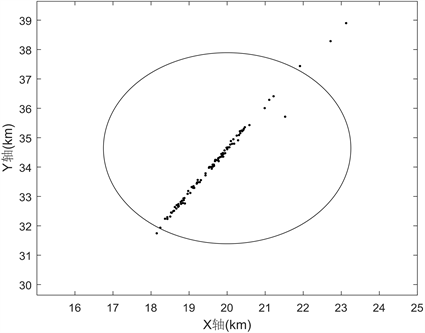Figure 5. Estimated location of single-station target (3)

4. 结论

 孙仲康, 郭福成, 冯道旺, 等.单站无源定位跟踪技术[M]. 北京: 国防工业出版社, 2008: 5-6.

 郭福成, 樊昀, 周一宇, 等. 空间电子侦察定位原理[M]. 北京: 国防工业出版社, 2012: 1-2.

 蒋伊琳, 刘梦楠, 郜丽鹏, 等. 运动多站无源时差/频差联合定位方法[J]. 系统工程与电子技术, 2019, 41(7): 1441-1449.

 田明辉, 马敏, 张文祎. 基于方位测量和速度估计的目标定位跟踪[J]. 太赫兹科学与电子信息学报, 2019, 17(1): 69-73.

 左燕, 陈志猛, 蔡立平. 基于约束总体最小二乘的单站DOA/TDOA联合误差校正与定位算法[J]. 电子信息学报, 2019, 41(6): 1317-1323.

 邢怀玺, 张宇晖, 陈游, 等. 基于MCIS技术的相位差变化率单站无源定位[J]. 航空学报, 2021, 41(3): 324278.

 邢怀玺, 吴华, 陈游. LMS预处理的相位差机载单站无源定位方法[J]. 空军工程大学学报(自然科学版), 2019, 20(6): 9-14.

 刘文跃, 彭世蕤, 王光学, 等. 基于差值定理的相位差变化率提取方法[J]. 雷达科学与技术, 2020, 18(4): 417-421.

 熊露, 谌剑, 毕晓文, 等. 基于迭代观测更新滤波的单站无源定位算法[J]. 水下无人系统学报, 2019, 27(4): 406-412.

 周桃云, 梁平元, 张怡. 一种新的可控粒子数无源定位滤波算法研究[J]. 弹箭与制导学报, 2015, 35(3): 157-161.

Top